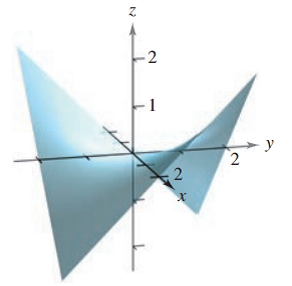Chapter 7.5, Problem 15E### Calculus: An Applied Approach (Min...

10th Edition
Ron Larson
ISBN: 9781305860919

#### Solutions

Chapter
Section### Calculus: An Applied Approach (Min...

10th Edition
Ron Larson
ISBN: 9781305860919
Textbook Problem
1 views

# Applying the Second-Partials Test In Exercises 1-18, find the relative extrema and saddle points of the function. See Examples f ( x , y ) = 1 2 x yTo determine

To calculate: The relative extrema and saddle points of the function f(xy)=12xy.

Explanation

Given Information:

The equation of the function is given as f(xy)=12xy.

The provided figure is,

Formula used:

To check the relative extrema and saddle points

d=fxx(a,b)fyy(a,b)[fxy(a,b)]2

Following steps to be followed:

Step 1: If d>0 and fxx(a,b)>0 then the function has a relative minimum at (a,b).

Step 2: If d>0 and fxx(a,b)<0 then the function has a relative minimum at (a,b)

Step 3: If d<0 then (a,b,f(a,b)) is a saddle point.

Step 4: The test gains no information when d=0.

Calculation:

Consider the given equation and differentiate with respect to x and y respectively,

f(x,y)=12xyfx(x,y)=12yfy(x,y)=12x

Now, fx(x,y) and fy(x,y) are defined for all points in the xy-plane

### Still sussing out bartleby?

Check out a sample textbook solution.

See a sample solution

#### The Solution to Your Study Problems

Bartleby provides explanations to thousands of textbook problems written by our experts, many with advanced degrees!

Get Started

#### In problems 1-16, solve each equation. 8. Solve

Mathematical Applications for the Management, Life, and Social Sciences

#### Find if , y = sin 2t.

Study Guide for Stewart's Single Variable Calculus: Early Transcendentals, 8th

#### True or False: converges.

Study Guide for Stewart's Multivariable Calculus, 8th